Next: Lorentz Boost Up: Lorentz Covariance Previous: Lorentz Covariance

# Lorentz Group

The form of a theory has to be invariant under a transformation from one inertial system to another. Consider two observers,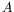and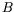, in different inertial systems. They describe the same physical event with their particular (different) space-time coordinates. Let the coordinates of the event be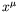for observerand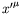for observer.

A Lorentz change of reference is a real linear transformation of the coordinates conserving the norm of the intervals between the different points in space-time. By means of the Lorentz transformation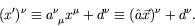(3.1)

The real vector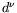represents a simple translation of the space-time axes. In what follows, we shall treat the translations separately and give the name Lorentz transformation to the homogeneous translations (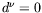). The group formed by all Lorentz transformations including translations is commonly called the inhomogeneous Lorentz group, or Poincaré group.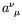is real (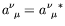) and depends only on the relative velocities and spatial orientations of reference frames.

The inverse transformation is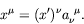(3.2)

The distance between two space-time points is invariant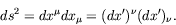(3.3)

To see this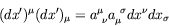(3.4)

and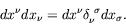(3.5)

Therefore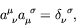(3.6)

which is the orthogonality relation for the Lorentz transformation.

The determinant is calculated using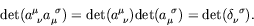(3.7)

Since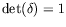,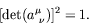(3.8)

Therefore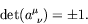(3.9)

For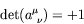(3.10)

we have a proper Lorentz transformation (rotations and boosts).

For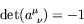(3.11)

we have an improper Lorentz transformation (discrete reflection either in space or in time). Including the improper Lorentz transformation of the reflection in space into the Lorentz group gives the orthochronous Lorentz group. If time reversal is also included, it is called the complete Lorentz group.

For an infinitesimal transformation we can write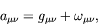(3.12)

where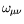is infinitesimal.

Since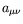is real,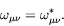(3.13)

Using the orthogonality relationship and only keeping terms to first order in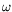, gives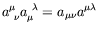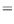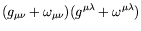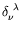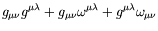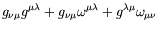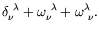(3.14)

Therefore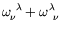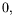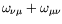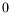(3.15)

andis an anti-symmetric tensor. A general Lorentz transformation thus has six parameters: three boost parameters and three rotation parameters.Next: Lorentz Boost Up: Lorentz Covariance Previous: Lorentz Covariance
Douglas M. Gingrich (gingrich@ ualberta.ca)
2004-03-18